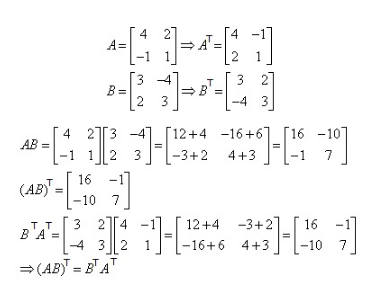# Given below A and B matrix then Show that (AB)=B^TA^T

Given below A and B matrix then Show that (AB)=B^TA^T